Courses

# Test: Congruence Of Triangles - 3

## 20 Questions MCQ Test Mathematics (Maths) Class 7 | Test: Congruence Of Triangles - 3

Description
This mock test of Test: Congruence Of Triangles - 3 for Class 7 helps you for every Class 7 entrance exam. This contains 20 Multiple Choice Questions for Class 7 Test: Congruence Of Triangles - 3 (mcq) to study with solutions a complete question bank. The solved questions answers in this Test: Congruence Of Triangles - 3 quiz give you a good mix of easy questions and tough questions. Class 7 students definitely take this Test: Congruence Of Triangles - 3 exercise for a better result in the exam. You can find other Test: Congruence Of Triangles - 3 extra questions, long questions & short questions for Class 7 on EduRev as well by searching above.
QUESTION: 1

### In ΔABC,AB = AC and AD is ⊥ to BC. State the property by which ΔADB = ΔADC.

Solution:

According to the question, the triangles formed are right angled triangles, as shown in the figure.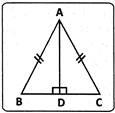QUESTION: 2

### Two students drew a line segment each. What is the condition for them to be congruent?

Solution:

Line segments of equal length are congruent.

QUESTION: 3

### In the given figure, if AD = BC and AD||BC,which of the following is true?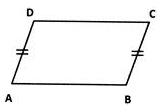Solution:

In the given figure,AD = BC,AC = AC,  and ∠DAC = ∠BCA
By S.A.S. theorem ΔABC≅ΔCDA
So, AB=DC.
(Corresponding parts of congruent triangles.)

QUESTION: 4

In ΔPQR and ΔXYZ, ∠P = 50o, XY = PQ, and XZ = PR. By which property are ΔXYZ and ΔPQR congruent?

Solution: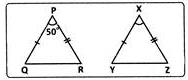In ΔPQR and ΔXYZ. ∠P = ∠X = 50o PQ = XY  and PR  =XZ.
∴ Δ PQR ≅ Δ XYZ (S.A.S. property)

QUESTION: 5

A: Two triangles are said to be congruent if two sides and an angle of one triangle are respectively equal to the two sides and an angle of the other.
R: Two triangles are congruent if two sides and the included angle of one are equal to the corresponding two sides and included angle of the other.
Given A & R, which of the following statements is correct?

Solution:

Including angle means the angle formed by two lines. This means that two sides of one triangle which are equal to two sides of other triangle have an including angle and only that angle should be equal.

QUESTION: 6

Two triangles, A PQR and ADEF are of the same size and shape. What can we conclude about them?

Solution:

Two triangles are congruent when they are of the same shape and size , if any one of them is made to superpose on the other , it will cover exactly .Since both are equal , they cannot be smaller or larger.

QUESTION: 7

Which of the following examines the congruence of plane figures?

Solution:
QUESTION: 8

Which of the following is a pair of congruent figures?

Solution:
QUESTION: 9

A In ΔABC and ΔPQR, AB=x cm, BC=y cmand CA=z cm. What are the measures of sides PQ, QR and RP of ΔPQR if ΔABC ≅ ΔABC ≅ ΔPQR?

Solution:

Corresponding parts of congruent triangles are equal.
So, PQ = AB = x cm, QR = BC = y cm and RP = CA = z cm.

QUESTION: 10

In the given figure, AD = CD and AB = CB.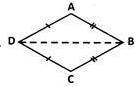What are the three pairs of equal parts?

Solution:

Corresponding sides in ΔABD and ΔCBD.

QUESTION: 11

In the given figure, AD = CD and AB = CB.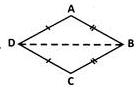Which of the following is the correct conclusion?

Solution:

Since ΔABD  and  ΔCBD  are  congruent, ∠ADB=∠CDB
(Corresponding parts of congruent triangles).⇒ BD bisects ∠ADC

QUESTION: 12

In the given figure, AB=AC and AD is the bisector of ∠BAC.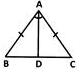Which among the following statements is true?

Solution:

We have AB=AC.Angles opposite to these sides will also be equal. So angles opposite to these sides are angle and angle c. So angle b and angle c are equal.

QUESTION: 13

In ΔABC and ΔDEF, AC = DF,AB = DE and BC=EF. By which property are ΔABC and ΔDEF congruent?

Solution:
QUESTION: 14

For the triangles ΔART and ΔPEN given, if S.A.S. criterion should be used given ∠T = ∠N what are the respective measures of PN and RT?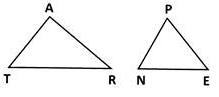Solution:
QUESTION: 15

Which of the following is an example of A.S.A. criterion of congruency for two triangles ∠ACB and ∠DEF?

Solution:
QUESTION: 16

In the figure given, AC = BD and ∠BAC = ∠CDB = 90o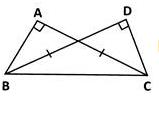If ΔABC = ΔDCB by R.H.S. property which of the following is required?

Solution:

BC is the hypotenuse of the given triangles. Whose measure must be known.

QUESTION: 17

Which of the following criterion does not exist?

Solution:

A.A.A. criterion does not exist.

QUESTION: 18

In two triangles, the three angles of one triangle are correspondingly equal to three angles of another triangle. Which of the following is a correct statement?

Solution:

If the three angles of a triangle are congruent to corresponding angles of the other, it is an enlarged copy of the triangle.

QUESTION: 19

ΔABC is congruent to ΔPQR under the correspondence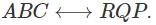Which part corresponds to PQ?

Solution: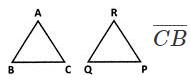corresponds to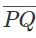QUESTION: 20

ΔABC is congruent to ΔPQR under the correspondence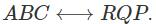Which part of corresponds to RP?

Solution: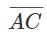corresponds to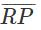[Note that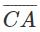corresponds to PR.]